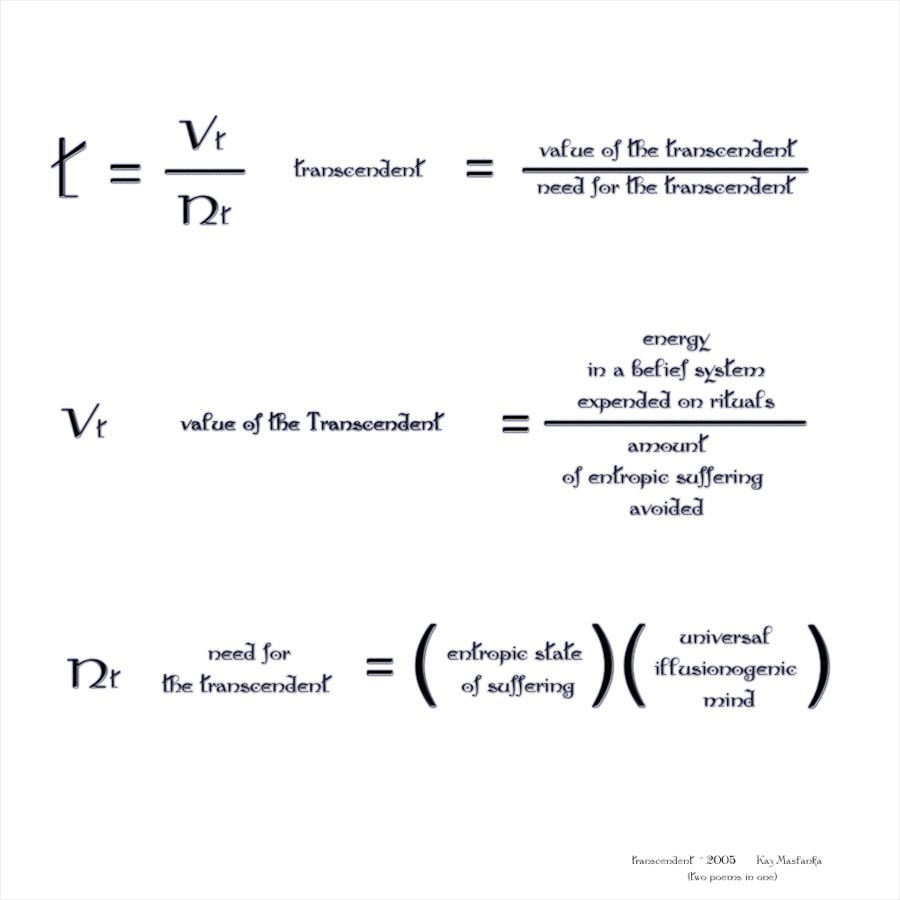# Poem one mathematically contains both poem two and poem three by variable substitution

## Poem one:

t = v/n

t = the transcendent

v = value of the transcendent

n = need for the transcendent

transcendent = value of the transcendent / need for the transcendent

## Poem two:

v = r/se

value of the Transcendent = energy in a belief system expended on rituals / The amount of entropic suffering avoided

n = m(es)

The need for the transcendent = (The universal illusionogenic mind)(the entropic state of suffering)

## combined equations:

t = r/se/m(es)

t = r/(se)(m)(es)

transcendent = ( energy in a belief system expended on rituals / ((amount of entropic suffering avoided )(The universal illusionogenic mind)(the entropic state of suffering))